Share

# RD Sharma solutions for Class 8 Mathematics chapter 19 - Visualising Shapes

## Mathematics for Class 8 by R D Sharma (2019-2020 Session)

#### RD Sharma Mathematics Class 8 by R D Sharma (2019-2020 Session)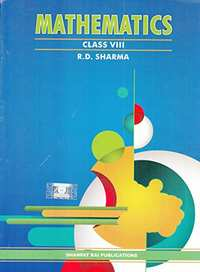## Chapter 19: Visualising Shapes

Ex. 19.10Ex. 19.20

#### Chapter 19: Visualising Shapes Exercise 19.10 solutions [Pages 9 - 10]

Ex. 19.10 | Q 1 | Page 9

What is the least number of planes that can enclose a solid? What is the name of the solid?

Ex. 19.10 | Q 2.1 | Page 9

Can a polyhedron have for its face 3 triangles?

Ex. 19.10 | Q 2.2 | Page 9

Can a polyhedron have for its face 4 triangles?

Ex. 19.10 | Q 2.3 | Page 9

Can a polyhedron have for its face a square and four triangles?

Ex. 19.10 | Q 3 | Page 9

Is it possible to have a polyhedron with any given number of faces?

Ex. 19.10 | Q 4 | Page 9

Is a square prism same as a cube?

Ex. 19.10 | Q 5 | Page 9

Can a polyhedron have 10 faces, 20 edges and 15 vertices?

Ex. 19.10 | Q 6.1 | Page 9

Verify Euler's formula for the following polyhedron: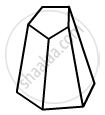Ex. 19.10 | Q 6.2 | Page 9

Verify Euler's formula for the following polyhedron:Ex. 19.10 | Q 6.3 | Page 9

Verify Euler's formula for the following polyhedron: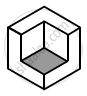Ex. 19.10 | Q 6.4 | Page 9

Verify Euler's formula for the following polyhedron: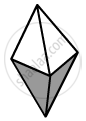Ex. 19.10 | Q 6.5 | Page 9

Verify Euler's formula for the following polyhedron: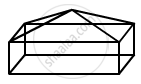Ex. 19.10 | Q 7 | Page 10

Using Euler's formula find the unknown:

 Faces ? 5 20 Vertices 6 ? 12 Edges 12 9 ?

#### Chapter 19: Visualising Shapes Exercise 19.20 solutions [Pages 12 - 14]

Ex. 19.20 | Q 1 | Page 12

Which among the following are nets for a cube?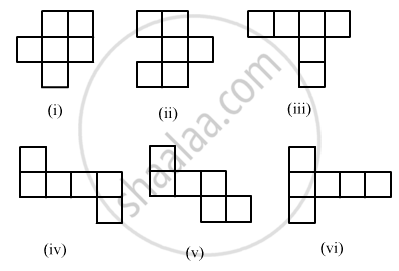Ex. 19.20 | Q 2.1 | Page 13

Name the polyhedron that can be made by folding net: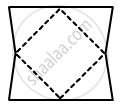Ex. 19.20 | Q 2.2 | Page 13

Name the polyhedron that can be made by folding net: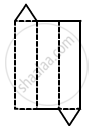Ex. 19.20 | Q 2.3 | Page 13

Name the polyhedron that can be made by folding net: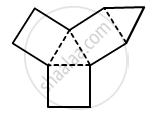Ex. 19.20 | Q 2.4 | Page 13

Name the polyhedron that can be made by folding net: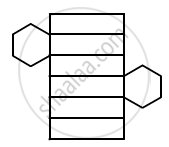Ex. 19.20 | Q 2.5 | Page 13

Name the polyhedron that can be made by folding net: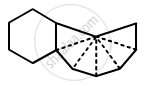Ex. 19.20 | Q 2.6 | Page 13

Name the polyhedron that can be made by folding net: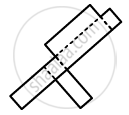Ex. 19.20 | Q 3 | Page 13

Dice are cubes where the numbers on the opposite faces must total 7. Which of the following are dice?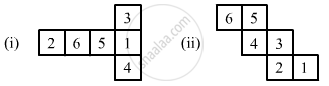Ex. 19.20 | Q 4.1 | Page 13

Draw net for the following polyhedron :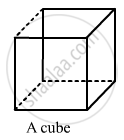Ex. 19.20 | Q 4.2 | Page 13

Draw net for the following polyhedron :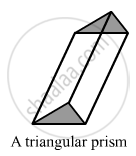Ex. 19.20 | Q 4.3 | Page 13

Draw net for the following polyhedron :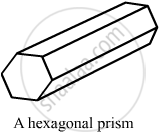Ex. 19.20 | Q 4.4 | Page 13

Draw net for the following polyhedron :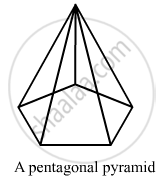Ex. 19.20 | Q 5 | Page 14

Match the following figure: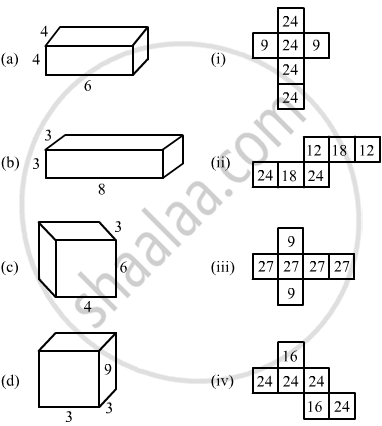## Chapter 19: Visualising Shapes

Ex. 19.10Ex. 19.20

#### RD Sharma Mathematics Class 8 by R D Sharma (2019-2020 Session)## RD Sharma solutions for Class 8 Mathematics chapter 19 - Visualising Shapes

RD Sharma solutions for Class 8 Maths chapter 19 (Visualising Shapes) include all questions with solution and detail explanation. This will clear students doubts about any question and improve application skills while preparing for board exams. The detailed, step-by-step solutions will help you understand the concepts better and clear your confusions, if any. Shaalaa.com has the CBSE Mathematics for Class 8 by R D Sharma (2019-2020 Session) solutions in a manner that help students grasp basic concepts better and faster.

Further, we at Shaalaa.com are providing such solutions so that students can prepare for written exams. RD Sharma textbook solutions can be a core help for self-study and acts as a perfect self-help guidance for students.

Concepts covered in Class 8 Mathematics chapter 19 Visualising Shapes are Views of 3D-shapes, Mapping Space Around Us, Faces, Edges and Vertices.

Using RD Sharma Class 8 solutions Visualising Shapes exercise by students are an easy way to prepare for the exams, as they involve solutions arranged chapter-wise also page wise. The questions involved in RD Sharma Solutions are important questions that can be asked in the final exam. Maximum students of CBSE Class 8 prefer RD Sharma Textbook Solutions to score more in exam.

Get the free view of chapter 19 Visualising Shapes Class 8 extra questions for Maths and can use Shaalaa.com to keep it handy for your exam preparation

S